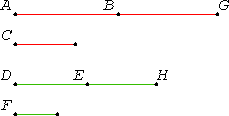# Proposition 24

If a first magnitude has to a second the same ratio as a third has to a fourth, and also a fifth has to the second the same ratio as a sixth to the fourth, then the sum of the first and fifth has to the second the same ratio as the sum of the third and sixth has to the fourth.

Let a first magnitude AB have to a second C the same ratio as a third DE has to a fourth F, and let also a fifth BG have to the second C the same ratio as a sixth EH has to the fourth F.

I say that the sum of the first and fifth, AG, has to the second C the same ratio as the sum of the third and sixth, DH, has to the fourth F.V.7.Cor

Since BG is to C as EH is to F, inversely, C is to BG as F is to EH.

V.22

Then, since AB is to C as DE is to F, and C is to BG as F is to EH, therefore, ex aequali, AB is to BG as DE is to EH.

V.18

And, since the magnitudes are proportional taken separately, they are also proportional taken jointly, therefore AG is to GB as DH is to HE.

V.22

But also BG is to C as EH is to F, therefore, ex aequali, AG is to C as DH is to F.

Therefore, if a first magnitude has to a second the same ratio as a third has to a fourth, and also a fifth has to the second the same ratio as a sixth to the fourth, then the sum of the first and fifth has to the second the same ratio as the sum of the third and sixth has to the fourth.

Q.E.D.

## Guide

This proposition says that if u : v = w : x and y : v = z : x, then (u + y) : v = (w + z) : x.

The argument goes as follows. Start by inverting the second proportion y : v = z : x to get v : y = x : z. Compound the first proportion with that to get u : y = w : z. Take that last proportion jointly to get (u + y) : y = (w + z) : z. Compound that with the second proportion to get (u + y) : v = (w + z) : x, which was the goal.

Although the proposition is stated using the antecedent terms of the proportions, by inversion it applies to the consequent terms as well.